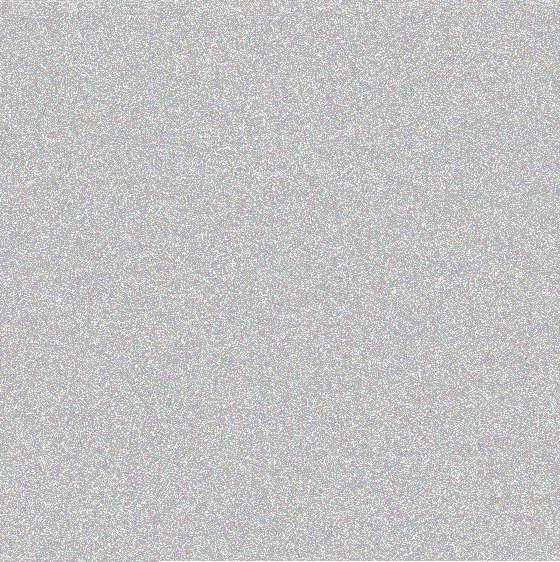# A Picture is worth how many words? Part 1

i was playing around with some imaging code, and started wondering what it would look like to create some images out of text. i have worked with stenography in the past, but this time i didn’t want to hide the text, i wanted to pack the image with it.

First things first, i need some text. Time to turn to trusty Project Gutenberg. i will be using the text of Moby Dick for this project.

``````with open('~/Desktop/pg2701.txt') as fp:
print len(words)
>>> 1257274``````

Now i need a couple of functions to help me get started. First, it doesn’t really matter what kind of dimensions my image has, but i would like it to be roughly square.

``````import math

def getDimensions(data, size=4):
''' Get a rough square based on the amount of data we have '''
bits = math.ceil(len(data) / float(size))
w = math.floor(math.sqrt(bits))
h = math.ceil(bits / w)
return (int(w), int(h))``````

i am also going to define a generator that will turn my data into a stream of data that will be able to be understood by PIL.

``````def toPixelStream(data, size=4):
''' Takes a string and turns it into a sequence of pixel data '''
start = 0
while start < len(data):
b = data[start:start + size]
if len(b) < size:
b = b + (' ' * (size - len(b)))
yield tuple([ord(i) for i in b])
start += size``````

Now i need to decide what image format to use. Here are some of my choices:

• PNG
• JPG
• GIF
• TIFF

JPG is out right away because it is a lossy format. There is no guarantee that any data we put into is going to come back out (i will show you an example in my next post).

GIF isn’t real well suited either because it is an indexed format, and thus limits the number of colors we can use to represent our data. (But… there is a way…)

That leaves PNG and TIFF. This time, i will use PNG.

Remember that each pixel in a PNG has four components: Red, Green, Blue, and Alpha. Each of those is one byte, so we will be able to shove four characters into each pixel. Let’s construct some image data!

``````imgdata = [i for i in toPixelStream(words)]
print len(imgdata)
>>> 314320
print imgdata[:4]
>>> [(239, 187, 191, 84), (104, 101, 32, 80), (114, 111, 106, 101), (99, 116, 32, 71)]``````

Now we have a list of tuples. Each tuple represents a pixel: (R, G, B, A), and each component of that pixel is the ord of a character in our text. All we have left is to write that out to a file.

``````from PIL import Image

img = Image.new('RGBA', getDimensions(words)))
img.putdata(imgdata)

fp = open('~/Desktop/pg2701.png', 'w')
img.save(fp)
fp.close()``````

To the Desktop!And there it is! The entire work of Moby Dick in an image!

But wait, you say. How can we know that our data survived? Ok, let’s try to pull our data back out of the image.

``````fp = open('~/Desktop/pg2701.png')
im = Image.open(fp)
newdata = im.getdata()
book = []
for pixel in newdata:
for component in pixel:
book.append(chr(component))
book = ''.join(book)
print book[:181]

>>> """The Project Gutenberg EBook of Moby Dick; or The Whale, by Herman Melville

This eBook is for the use of anyone anywhere at no cost and with
almost no restrictions whatsoever."""``````

It worked!